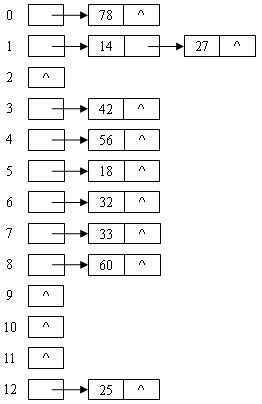# 2008秋-计算机软件基础-第四章习题参考答案 P131 ，习题4，5

Author: Eman Lee

P131，第4 参考答案

1）查找e的过程

 a b c d e f g h Low=1 Mid=4 High=8 a b c d e f g h Low=5 Mid=6 High=8 a b c d e f g h Low=5 Mid=5 High=5 查找成功

2）查找f的过程

 a b c d e f g h Low=1 Mid=4 High=8 a b c d e f g h Low=5 Mid=6 High=8 查找成功

3）查找h的过程

 a b c d e f g h Low=1 Mid=4 High=8 a b c d e f g h Low=5 Mid=6 High=8 a b c d e f g h Low=7 Mid=7 High=8 a b c d e f g h 查找成功 Low=8 Mid=8 High=8

P131，第5 参考答案

 关键字Ki 18 25 14 56 78 33 27 32 60 42 哈希地址 5 12 1 4 0 7 1 6 8 3

（1）       线性探测再散列， H(Ki)=(H(Ki)+di)%m, di=1,2,3,….m-1

 哈希地址 0 1 2 3 4 5 6 7 8 9 10 11 12 关键字Ki 78 14 27 42 56 18 32 33 60 25 比较次数 1 1 2 1 1 1 1 1 1 1

（2）       二次探测再散列, H(Ki)=(H(Ki)+di)%m, di=1*1,-1*1,2*2,-2*2,…..(m/2)*(m/2), -.(m/2)*(m/2)

 哈希地址 0 1 2 3 4 5 6 7 8 9 10 11 12 关键字Ki 78 14 27 42 56 18 32 33 60 25 比较次数 1 1 2 1 1 1 1 1 1 1

3）链地址法posted @ 2007-10-20 22:57 emanlee 阅读(...) 评论(...) 编辑 收藏